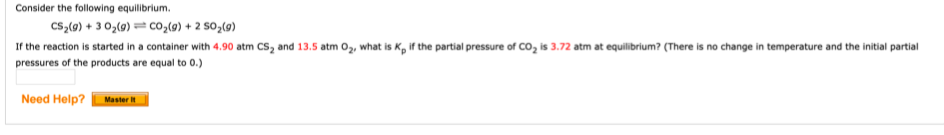# Consider the following equilibrium. CS2(g) + 3 02(g) - CO2(g) + 2 S02(g) If the reaction is started in a container with 4.90 atm CS2 and 13.5 atm 02, what is Kp if the partial pressure of CO2 is 3.72 atm at equilibrium? (There is no change in temperature and the initial partial pressures of the products are equal to 0.)